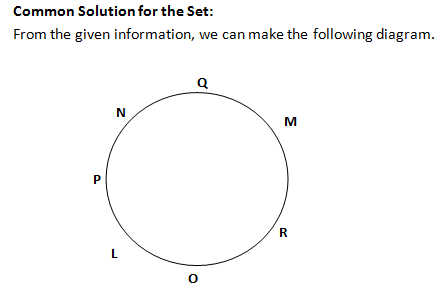### Logical Reasoning topics: Different types of Logical Reasoning topics and questions that you should solve for exams

Logical reasoning topics cover a vast variety of things. The types of sets which fall under logical reasoning topics are:
– Seating arrangement questions
– Circular arrangement questions
– Direction based questions
– Sets based on family relations
– Analytical reasoning sets
– Other miscellaneous kinds which combine mathematics and Data interpretation
with these topics.
So, as far as the breadth of topics goes, you need to cover a wide variety of logical reasoning topics to perfect your skills for this area.

Directions for the question set: (1 to 3)
Study the following information to answer the given questions:
L, M, N, O, P, Q and R are sitting around a circle facing the centre. O is sitting between L and R. Q is second to the right of R and P is second to the right of Q. N is not an immediate neighbour of R.

Question 1: Which of the following is not correct?
(a) R is second to the right of L
(b) M is second to the left of N
(c) L sits exactly between O and P
(d) P and N are immediate neighbours
(e) P sits to the opposite of N

Question 2: How many persons are seated between L and Q if we count anticlockwise from L to Q?
(a) One
(b) Two
(c) Three
(d) Four
(e) More than four

Question 3: Who is to the immediate left of P?
(a) L
(b) N
(c) M
(d) O
(e) None of these

Directions for question (4-5):

Question 4: Four of the following five are alike in a certain way based on their seating positions in the above arrangement and so form a group. Which is the one that does not belong to the group?
(a) QPN
(b) MOR
(c) LRO
(d) RQM
(e) ROL

Question 5: Which is the one that does not belong to the group?
(a) LP
(b) RM
(c) MQ
(d) NP
(e) OR

### Answers and Explanations: Click the down arrow to expandAnswer 1: (e) P sits by the side of N Hence it is incorrect. Correct option is (e).

Answer 2: (c) There are 3 people in the anti-clockwise direction between L and Q. Correct option is (c).

Answer 3: (b) N is to the immediate left of P. Correct option is (b).

Answer 4: (e) Unlike all other options ROL are in order in clockwise direction. Correct option is (e).

Answer 5: (a) In all the options except the 1st, the 2nd person is sitting to the right of the first person. Hence, correct option is (a).

Extra tips for Logical Reasoning Topics:
• Make sure you expose yourself to as many logical reasoning topics as you can.
• Once you begin to identify the different types of questions possible here, you can clearly identify the approach you need to solve these questions.
• Learning is all about having the right resources. Use the content available on Wordpandit and use the relevant resources in order to expand your knowledge for logical reasoning topics.
• Make sure you practice regularly and try to learn the different approaches for these questions.